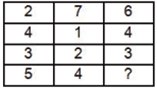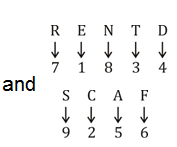# SSC Reasoning Ability Practice Questions (Day-56)

Dear Aspirants, The SSC Exams are going on at national level. Millions of candidates have eagerly prepared for the ongoing SSC exams such as CGL, CHSL, MTS, CPO, JE etc. SSC exams are usually conducted by the Staff Selection Commission to recruit the eligible candidates for various Central government departments. Mostly the selection process for all the SSC exams consists of the single or double phase of written examination based on the examination. Due to that, the written exam consisted of a certain syllabus and exam pattern. By following those regulations, the written exam has been conducted through an online mode. Based on the latest updated exam pattern and syllabus, here we have drafted the Reasoning Ability questions on a daily basis. So aspirants start your practice session with us and mould your skill in a perfect shape.

Start Quiz

1) Present age of a father is 3 times that of his son. After 10 years the son’s age will be 5 times of Raman’s present age. If Raman celebrated his thirdbirthday 2 years ago, then what is the present age (in years) of father?

(a) 45

(b) 40

(c) 36

(d) 39

Direction (2-3): In the following question, select the word which cannot be formed using the letters of the given word.

2) Precipitation

(a) Reaction

(b) Patient

(c) Reacts

(d) Petition

3) Imprisonment

(a) Prison

(b) Sonnet

(c) Impression

(d) Moment

4) In a certain code language, “BALL” is written as “27” and “CANE” is written as “23”. How is “YELL” written in that code language?

(a) 50

(b) 39

(c) 54

(d) 61

5) In a certain code language, “RENTED” is written as “718314” and “SCARF” is written as “92576”. How is “CARTED” written in that codelanguage?

(a) 257314

(b) 962514

(c) 237614

(d) 759613

6) In the following question, by using which mathematical operators will the expression become correct?

7 ?4 ?5 ?165 ? 5

(a) +, +, ÷ and =

(b) ×, ×, = and +

(c) ×, ÷, = and ÷

(d) ×, +, = and ÷

7) In the following question, correct the equation by interchanging two signs.

24 × 8 ÷ 9 + 9 – 10 = 26

(a) ÷ and –

(b) ÷ and ×

(c) × and –

(d) × and +

8) If 21 (49) 14 and 159 (169) 146, then what is the value of ‘A’ in 56 (A) 44?

(a) 144

(b) 121

(c) 225

(d) 256

9) If 23 # 43 @ 33 = 45 and 33 # 53 @ 43 = 88, then 43 # 23 @ 13= ?

(a) 48

(b) 71

(c) 56

(d) 65

Direction (10): In the following question, select the number which can be placed at the sign of question mark (?) from the given alterantives.

10)(a) 1

(b) 2

(c) 3

(d) 4

Father = 3 × son

After 10 years,

Son + 10 = 5 × Raman

Now, Raman’s present age = 3 + 2 = 5

∴ Son + 10 = 5 × 5 = 25

∴ Son = 15

∴ Present age of Father = 3 × 15 = 45 years.

Reacts ; There is no ‘s’ in the given word.

Impression ; There is no double ‘S’ in the given word.

BALL = 2 + 1 + 12 + 12 = 27

CANE = 3 + 1 + 14 + 5 = 23

YELL = 25 + 5 + 12 + 12 = 54∴ CARTED → 257314

7 × 4 + 5 = 165 ÷ 5

⇒ 28 × 5 = 33

⇒ 33 = 33

24 × 8 ÷ 9 + 9 – 10 = 26

⇒ 24 ÷ 8 × 9 + 9 – 10 = 26

⇒ 3 × 9 + 9 – 10 = 26

⇒ 27 + 9 – 10 = 26

⇒ 36 – 10 = 26

⇒ 26 = 26

(21 – 14)2 = 49

(159 –146)2 = 169

(56 – 44)2 =144

23 + 43 – 33 = 45

33 + 53 – 43 = 88

43 + 23 – 13 = 71

2 + 4 + 3 + 5 = 14

7 + 1 + 2 + 4 = 14

6 + 4 + 3 + ? = 14

?=1

 Check Here to View SSC CGL / CHSL 2021 Reasoning Ability Questions Day – 55 Day – 54 Day – 53